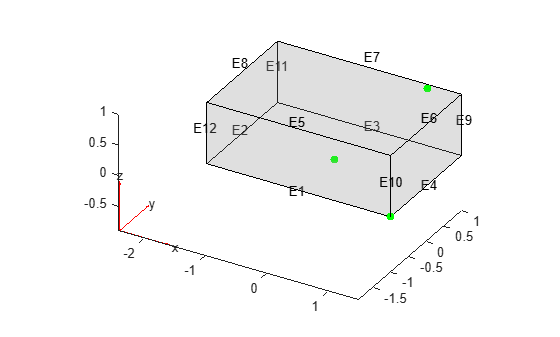nearestEdge

Find edges nearest to specified point

Description

example

EdgeID = nearestEdge(g,Coords) finds edges nearest to the point with the coordinates Coords.

Examples

collapse all

Find edges of a block nearest to the specified points.

Create a block geometry.

gm = multicuboid(3,2,1)
gm =
DiscreteGeometry with properties:

NumCells: 1
NumFaces: 6
NumEdges: 12
NumVertices: 8
Vertices: [8x3 double]

Plot the geometry with the edge labels. Add the points with the coordinates (0 0 0), (1 0.9 1), and (1.5 -1 0) to the plot.

pdegplot(gm,'EdgeLabels','on','FaceAlpha',0.2)
hold on
scatter3([0 1 1.5],[0 0.9 -1],[0 1 0],'filled','MarkerFaceColor','g')Find edges closest to the points with the coordinates (0 0 0), (1 0.9 1), and (1.5 -1 0). If several edges are equally close (within the tolerance) to the point, nearestEdge returns the ID of one of the edges.

edgeIDs = nearestEdge(gm,[0 0 0; 1 0.9 1; 1.5 -1 0])
edgeIDs = 1×3

1     7     4

Find edges of the L-shaped membrane nearest to the specified points.

Create a model and include this geometry. The geometry of the L-shaped membrane is described in the file lshapeg.

model = createpde();
gm = geometryFromEdges(model,@lshapeg)
gm =
AnalyticGeometry with properties:

NumCells: 0
NumFaces: 3
NumEdges: 10
NumVertices: 8
Vertices: [8x2 double]

Plot the geometry with the edge labels. Add the points with the coordinates (0 0), (0.1 0.2), and (-0.5 0.5) to the plot.

pdegplot(gm,'EdgeLabels','on')
hold on
scatter([0 0.1 -0.5],[0 0.2 0.5],'filled')Find edges closest to the points with the coordinates (0 0), (0.1 0.2), and (-0.5 0.5). If several edges are equally close (within the tolerance) to the point, nearestEdge returns the ID of one of the edges.

edgeIDs = nearestEdge(gm,[0 0; 0.1 0.2; -0.5 0.5])
edgeIDs = 1×3

7    10    10

Input Arguments

collapse all

Geometry, specified as a DiscreteGeometry or AnalyticGeometry object.

Coordinates of the point, specified as a vector of 2 or 3 elements for a 2-D or 3-D geometry, respectively.

Data Types: double

Output Arguments

collapse all

IDs of edges nearest to the specified point, returned as a positive number or a vector of positive numbers.